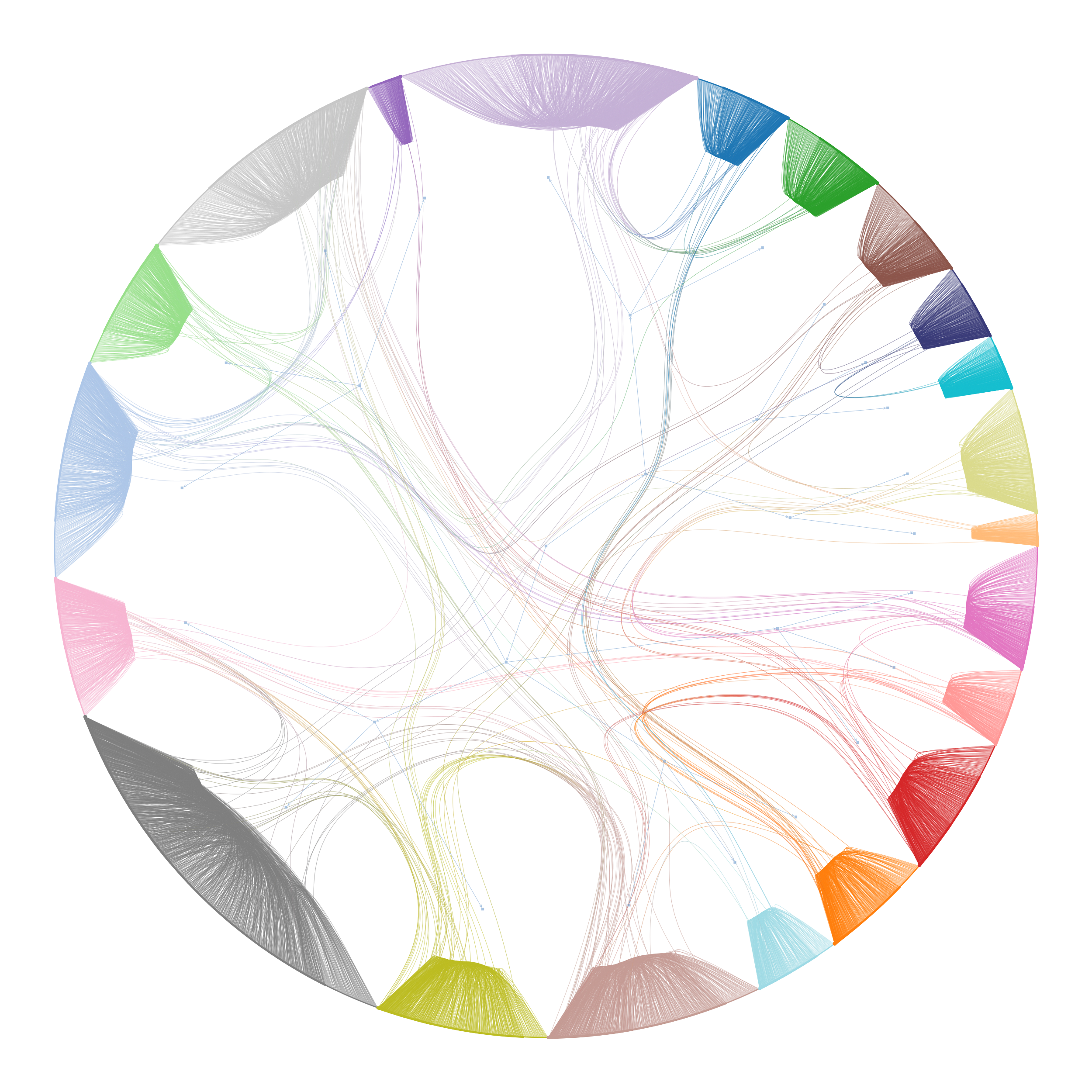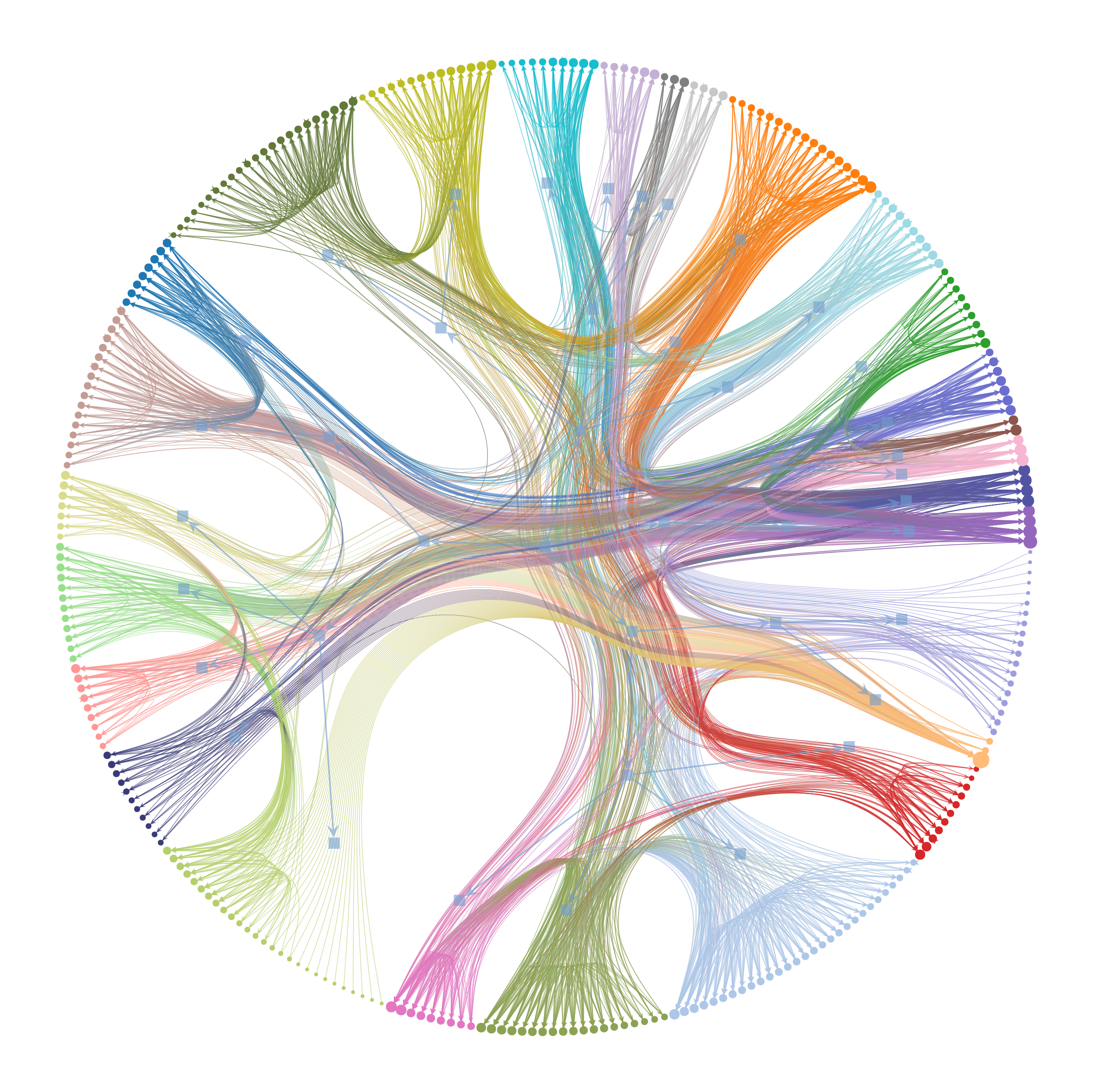# graph_tool.inference.minimize_nested_blockmodel_dl#

graph_tool.inference.minimize_nested_blockmodel_dl(g, init_bs=None, state=<class 'graph_tool.inference.nested_blockmodel.NestedBlockState'>, state_args={}, multilevel_mcmc_args={})[source]#

Fit the nested stochastic block model, by minimizing its description length using an agglomerative heuristic.

Parameters:
gGraph

The graph.

init_bsiterable of iterable of ints (optional, default: None)

Initial hierarchical partition.

B_minint (optional, default: 1)

The minimum number of blocks.

B_maxint (optional, default: numpy.iinfo(numpy.int64).max)

The maximum number of blocks.

b_minVertexPropertyMap (optional, default: None)

The partition to be used with the minimum number of blocks.

b_maxVertexPropertyMap (optional, default: None)

The partition to be used with the maximum number of blocks.

stateSBM state class (optional, default: NestedBlockState)

Type of model that will be used.

state_argsdict (optional, default: {})

Arguments to be passed to appropriate state constructor (e.g. BlockState)

multilevel_mcmc_argsdict (optional, default: {})

Arguments to be passed to multilevel_mcmc_sweep().

Returns:
min_statetype given by parameter state

State with minimum description length.

Notes

This function is a convenience wrapper around multilevel_mcmc_sweep().

See [peixoto-hierarchical-2014] for details on the algorithm.

This algorithm has a complexity of $$O(E \ln^2 V)$$, where $$E$$ and $$V$$ are the number of edges and nodes in the network, respectively.

References

Tiago P. Peixoto, “Hierarchical block structures and high-resolution model selection in large networks “, Phys. Rev. X 4, 011047 (2014), DOI: 10.1103/PhysRevX.4.011047 [sci-hub, @tor], arXiv: 1310.4377.

Examples

>>> g = gt.collection.data["power"]
>>> state = gt.minimize_nested_blockmodel_dl(g)
>>> state.draw(output="power_nested_mdl.pdf")
(...)Hierarchical Block partition of a power-grid network, which minimizes the description length of the network according to the nested (degree-corrected) stochastic blockmodel.#
>>> g = gt.collection.data["celegansneural"]
>>> state = gt.minimize_nested_blockmodel_dl(g, state_args=dict(overlap=True))
>>> state.draw(output="celegans_nested_mdl_overlap.pdf")
(...)Overlapping block partition of the C. elegans neural network, which minimizes the description length of the network according to the nested overlapping degree-corrected stochastic blockmodel.#